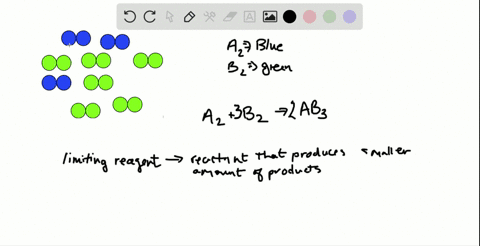Black Friday is Here! Start Your Numerade Subscription for 50% Off!Join Today### The scene below represents a mixture of A2 and B2…

04:09University of Maine
Problem 63

# What does the term stoichiometrically equivalent molar ratio mean, and how is it applied in solving problems?

## Discussion

You must be signed in to discuss.

## Video Transcript

story Kim magically equivalent ratios described that a specific amount of one substance in a chemical reaction will react with or produce specific amount of another substance. The's equivalence is are shown in what are called mole ratios were store Cheah magically equivalent ratios, and these come from the balanced equation. So if, for example, we have a reaction in which magnesium reacts with oxygen to form magnesium oxide, the balanced equation tells us we need to magnesium and to magnesium oxides. We can write thes as more ratios or strike you metric Lee equivalent ratios. This tells us that if there are two moles of magnesium will react with one mole of oxygen. One mole of oxygen will produce two moles of magnesium oxide and two moles of magnesium will produce two moles, a magnesium oxide. We can write thes as conversion factors or equivalency statements. Two moles. MGI equals one more, 02 or as a conversion factor, two moles mg over one more. Oh too. And these conversion factors can be used to convert quantities between different substances in the chemical reaction

Problem 4